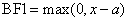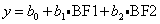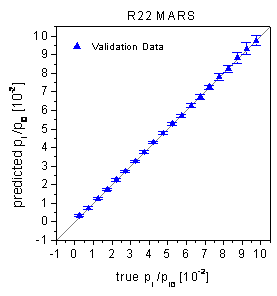Home News About Me Ph. D. Thesis Abstract Table of Contents 1. Introduction 2. Theory  Fundamentals of the Multivariate Data Analysis 3. Theory  Quantification of the Refrigerants R22 and R134a: Part I 4. Experiments, Setups and Data Sets 5. Results  Kinetic Measurements 6. Results  Multivariate Calibrations 6.1. PLS Calibration 6.2. Box-Cox Transformation + PLS 6.3. INLR 6.4. QPLS 6.5. CART 6.6. Model Trees 6.7. MARS 6.8. Neural Networks 6.9. PCA-NN 6.10. Neural Networks and Pruning 6.11. Conclusions 7. Results  Genetic Algorithm Framework 8. Results  Growing Neural Network Framework 9. Results  All Data Sets 10. Results  Various Aspects of the Frameworks and Measurements 11. Summary and Outlook 12. References 13. Acknowledgements Publications Research Tutorials Downloads and Links Contact Search Site Map Print this Page## 6.7.   MARS

The multivariate adaptive regression splines (MARS) were introduced by Friedman , as a multivariate nonparametric regression procedure. The MARS procedure fits separate splines, which are also called basis functions, to distinct intervals of the input variables. The basis functions have the general form:(31)

with BF1 as basis function, x as input variable and a as so-called knot. The transformation of the input variable is nonlinear, although the basis functions are piecewise linear. A regression using two basis functions can be described by:(32)

with y as response variable and b0, b1 and b1 as regression coefficients. Additionally, interactions up to a prescribed degree are also possible by the multiplication of two basis functions. The variables, the interactions and the locations of the knots are all found by a brute force approach and the regression coefficients are determined by a least squares procedure. The optimal model is found by a two-step algorithm similar to the CART principle. First, a model is grown by adding basis functions until an overfitting occurs. In the second phase, basis functions are deleted (pruned) until an optimal balance between overfitting and underfitting measured by the generalized crossvalidation error (GCV) has been reached for N samples and M basis functions:(33)

DOF (M) represents the degrees of freedom used by the basis functions. For linear regressions, DOF (M) is usually set to M. Increasing DOF prefers smaller models.

The MARS principle was applied to the data set of the refrigerants. The models for R22 and R134a were built by the use of the calibration data. Thereby the optimal DOF was determined by a 10-fold crossvalidation implemented in the MARS package . The degree of allowed interaction was systematically varied whereby the optimum for the crossvalidated calibration data was found allowing second order interactions.

For R22, the optimal MARS model contained 43 basis functions forming 3 additive and 27 interaction effects. In total 20 variables were used whereby the importance of the variables is shown in figure 41 measured by the relative amount of the reduction of the GCV by the corresponding variable. For R134a, the optimal model contained 43 basis functions forming 7 additive and 24 interaction effects. The relative importance of the 21 variables used by the model is also shown in figure 41. It is obvious that for both models the relative importance of the variables is very similar with the important variables forming two blocks after the beginning of exposure to analyte and after the end of exposure to analyte (>60 s). These blocks are similar to the blocks built by the CART, but in contrast to the CART both blocks are used for both analytes.figure 41:  Relative importance of the variables for the 2 MARS models measured by the reduction of the GCV.

According to table 2 the predictions of the calibration data are very promising with relative RMSE of 1.46% for R22 and 2.27% for R134a. The prediction errors of the validation data are significantly worse with 2.96% for R22 and 3.71% for R134a. The rather high numbers of basis functions used for models seem to overfit the calibration data. The true-predicted plots of the validation data in figure 42 demonstrate that the MARS deal well with the nonlinearities in the data and no significant bias of the predictions can be observed in agreement with the Wald-Wolfowitz Runs test and the Durbin-Watson statistics.figure 42:  True-predicted plots of the MARS for the validation data.

 Page 96 © Frank Dieterle, 03.03.2019